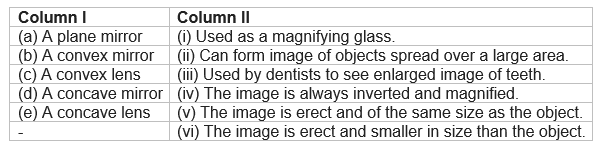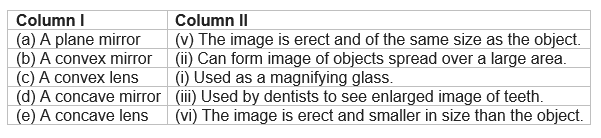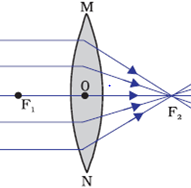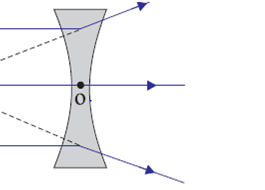# NCERT Solutions for Class 7 Science Chapter 15: Light

In this page we have NCERT Solutions for Class 7 Science Chapter 15: Light . Hope you like them and do not forget to like , social share and comment at the end of the page.

Question 1
Fill in the blanks:
(a) An image that cannot be obtained on a screen is called ____________.
(b) Image formed by a convex __________ is always virtual and smaller in size.
(c) An image formed by a __________ mirror is always of the same size as that of the object.
(d) An image which can be obtained on a screen is called a _________ image.
(e) An image formed by a concave ___________ cannot be obtained on a screen.
(a) virtual image.
(b) mirror
(c) plane
(d) real
(e) lens

Question 2
Mark T if the statement is true and F if it is false:
(a) We can obtain an enlarged and erect image by a convex mirror. (T/F)
(b) A concave lens always form a virtual image. (T/F)
(c) We can obtain a real, enlarged and inverted image by a concave mirror. (T/F)
(d) A real image cannot be obtained on a screen. (T/F)
(e) A concave mirror always form a real image. (T/F)
(a) F
(b) T
(c) T
(d) F
(e) F

Question 3
Match the items given in Column I with one or more items of Column II.Question 4
State the characteristics of the image formed by a plane mirror.
Characteristics of the image formed by a plane mirror are
(i) The image formed is virtual and erected
(ii) The image is laterally inverted. The left side appears on the right side of the image
(iii) It is of the same size as the object.
(iv) The image is situated at the same distance from the mirror as the object.

Question 5
Find out the letters of English alphabet or any other language known to you in which the image formed in a plane mirror appears exactly like the letter itself. Discuss your findings.
A, H, I, M, O, T, U, V, W, X, Y are the letters of English alphabet in which the image formed in a plane mirror appears exactly like the letter itself. These letter are vertically symmetric i.e., Left side is exactly same as right side.

Question 6
What is a virtual image? Give one situation where a virtual image is formed.
The image which cannot be formed or obtained on the screen is called virtual image.
When we stand in front of our dressing table mirror, we use to see our virtual image. The virtual image is formed in case of plane and convex mirror.

Question 7
State two differences between a convex and a concave lens.
 Convex lens Concave lens Convex lens converges the light falling on it.Concave lens diverges the light falling on it.Convex lens is thicker in the middle. Concave lens is thinner in the middle.

Question 8
Give one use each of a concave and a convex mirror.
Concave mirror are used by dentist
As concave mirror forms large images therefore it is used by dentists to see enlarged image of teeth.
Convex mirror used in rear view mirror in Vehicles

Convex mirror forms diminished image therefore it used as rear view mirror in vehicles

Question 9
Which type of mirror can form a real image?
Concave mirror.

Question 10
Which type of lens forms always a virtual image?
Concave lens forms always a virtual image.

Question 11
A virtual image larger than the object can be produced by a
(i) concave lens
(ii) concave mirror
(iii) convex mirror
(iv) plane mirror
(ii) concave mirror can produce a virtual image larger than the object.

Question 12
David is observing his image in a plane mirror. The distance between the mirror and his image is 4 m. If he moves 1 m towards the mirror, then the distance between David and his image will be
(i) 3 m
(ii) 5 m (iii) 6 m
(iv) 8 m
(iii) 6 m

Question 13
The rear view mirror of a car is a plane mirror. A driver is reversing his car at a speed of 2 m/s. The driver sees in his rear view mirror the image of a truck parked behind his car. The speed at which the image of the truck appears to approach the driver will be
(i) 1 m/s
(ii) 2 m/s
(iii) 4 m/s
(iv) 8 m/s
(iii) 4 m/s
In case of plane mirror, the distance is always doubled therefore the speed is in case between the image and the object. So, when driver is reversing his car at a speed of 2 m/s, then the image is also coming closer to the mirror by 2m/s. Thus, it seems that the image of the truck appears to approach the driver at 4 m/s

• Notes
• NCERT Solutions
• Assignments